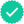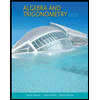#### Related questions#### Concept explainers

Question

Why is 00 an indeterminate form while 10 is not? Explain this in a few steps.
(a) Recall that a 0 0 indeterminate form means that both the numerator and the denominator approach zero (but never actually equal zero!). In terms of the base and the exponent, what does the indeterminate form 00 mean?

(b)Recall that a 0 0 indeterminate form could result in anything from (−∞,∞), if the limit exists. Why is this? Neither the numerator nor the denominator ever get to zero exactly. If the numerator approaches zero faster, the result is zero. If the denominator approaches zero faster, the result is ∞. If one of the terms approach zero from the left, the result could be negative.
Assuming the limit exists, how could the result of any 00 limit be between 0 and 1? Assume the base is always positive.

(c) (Extra credit) Actually the range of possible results for a limit with a 00 indeterminate form is anywhere from 0 and ∞. Provide a speciﬁc example of a limit with the form 00 where the result is larger than 1.

Expert SolutionKnowledge BoosterRecommended textbooks for you
•Algebra & Trigonometry with Analytic Geometry
Algebra
ISBN:9781133382119
Author:Swokowski
Publisher:CengageAlgebra and Trigonometry (MindTap Course List)
Algebra
ISBN:9781305071742
Author:James Stewart, Lothar Redlin, Saleem Watson
Publisher:Cengage Learning
•Algebra & Trigonometry with Analytic Geometry
Algebra
ISBN:9781133382119
Author:Swokowski
Publisher:CengageAlgebra and Trigonometry (MindTap Course List)
Algebra
ISBN:9781305071742
Author:James Stewart, Lothar Redlin, Saleem Watson
Publisher:Cengage Learning